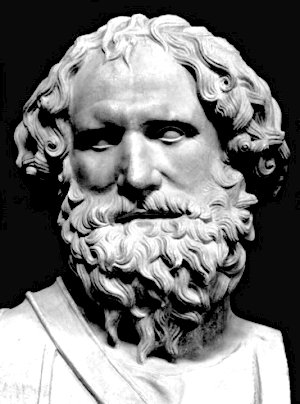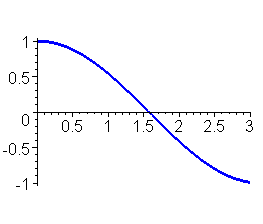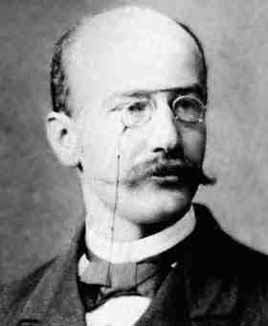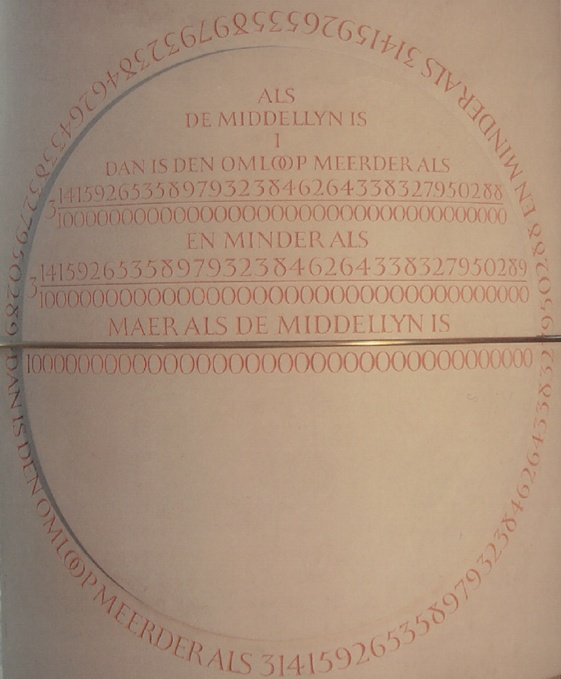Archimedes' constant p

p = 3.1415926535897932384626433832795028841971693993751...

And he made a molten sea, ten cubits from the one brim to the other;
it was round all about ... and a line ot thirty cubits did compass it round about.

- Bible, 1 Kings 7:23

This mysterious 3.141592..., which comes in at every door and window, and down every chimney.
- Augustus De Morgan (1806-1871)

## Definitions

### Geometrical definition

The constant p (Greek letter pi) is, classically, defined as the ratio of the circumference p of a circle to its diameter d:
 p = pd = 2pr

and, as proved by Archimedes of Syracuse (287-212 BC) in his famous Measurement of a Circle, the same constant is also the ratio of the area A enclosed by the circle to the square of its radius r:

 A = pr2.### Analytical definition

In 1934, the German mathematician Edmund Landau (1877-1938) gave a new and analytical definition based on the cosine function
 cos t = 1 - t2 2! + t4 4! - t6 6! + ....

Obviously cos 0  = 1 and cos 2 < -1/3 (take the 3 first terms of the sequence), from the continuity of the cosine function, the equation cos t = 0 has roots. If t1is the smallest positive root of this equation, p is defined as 2t1 . Several variants based on similar trigonometric equations are also possible.
Mathematicians prefer such definitions that avoid to fully define Euclidean geometry as well as an integration theory required to compute length or area.cosine function

## Notation

The first use of the symbol p to represent the ratio of the circumference to the diameter is given in Synopsis Palmariorum Mathseos by the Welsh mathematician William Jones (1675-1749) in 1706. It was probably inspired by the greek word for circumference: perijeria (peripheria). Later and progressively, this notation was popularized in his publications by the Swiss Leonhard Euler (1707-1783) .
p is also known as Archimedes' constant or Ludolph's number.

 In 1761, Johann Heinrich Lambert (1728-1777) was the first to prove that the number p is an irrational number . Adrien-Marie Legendre (1752-1833) also proved, in 1794, that p2 is irrational.It was not before the year 1882 that Ferdinand von Lindemann (1852-1939) succeeded to prove that p is a transcendental number  and therefore is not the root of any integer polynomials. With this last result, it was consequently established that the famous and old problem of squaring the circle with a ruler and a compass is insoluble.LambertLindemann

A simplified proof of the irrationality of p can be found in Irrationality proofs.

## Historical periodsvan Ceulen restored tombstone The history of the constant p is often divided into periods. The first period, or geometric period, began long ago with the Egyptians, Babylonians and Greeks determinations of the ratio of the circumference to its diameter and extended up to the beginning of the seventeenth century with Ludolph van Ceulen (1540-1610) 35 decimal places great calculation. It was the period of the geometricians and polygons computations.  The geometric period  The second period, or classic period, was the time of analytical developments, many infinite series or products were discovered to compute p. It started, around 1650, with the works of John Wallis (1616-1703), William Brouncker (1620-1684), James Gregory (1638-1675), Isaac Newton (1643-1727) and went up to the first electronic computation of p. A special chapter is devoted to the Indian mathematician Srinivasa Ramanujan (1887-1920) who found extraordinary series at the beginning of the twentieth century.  The classic period  The third period is recent and started around 1970 with the work of Richard Brent and Eugène Salamin who used previous results by Gauss and Legendre on the Arithmetic-Geometric Mean (AGM) to find a new kind of expression for p. Other similar results, discovered by Jonathan and Peter Borwein, made the computation of billions digits of p reachable.  Ramanujan series  Pi and AGM

## References


L. Euler, Introduction à l'analyse infinitésimale, (french traduction by Labey), Barrois, ainé, Librairie, (original 1748, traduction 1796), vol. 1

W. Jones, Synopsis Palmariorum Mathseos, London, (1706), p. 243 & p. 263

K. Knopp, Theory and application of infinite series, Blackie & Son, London, (1951)

J.H. Lambert, Mémoire sur quelques propriétés remarquables des quantités transcendentes circulaires et logarithmiques, Histoire de l'Académie Royale des Sciences et des Belles-Lettres der Berlin, (1761), pp. 265-276

F. Lindemann, Ueber die Zahl p, Mathematische Annalen, (1882), pp. 213-225

Back to Numbers, Constants and Computation